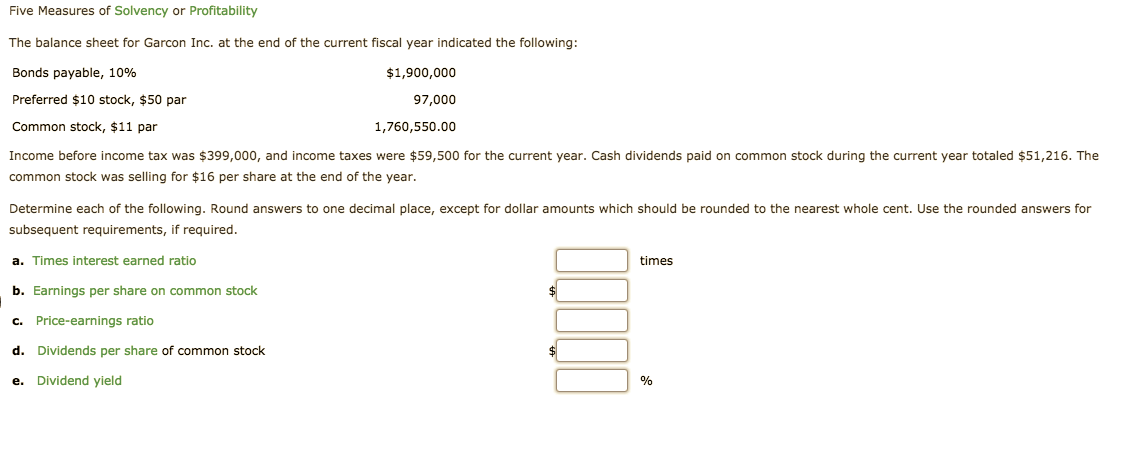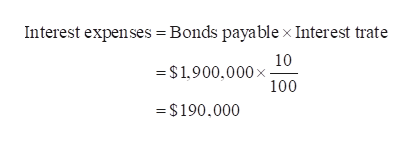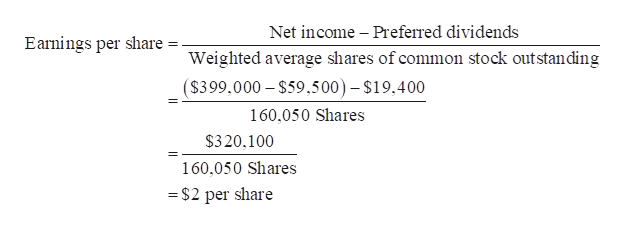Five Measures of Solvency or ProfitabilityThe balance sheet for Garcon Inc. at the end of the current fiscal year indicated the following:Bonds payable, 10%\$1,900,000Preferred \$10 stock, \$50 par97,000Common stock, \$11 par1,760,550.00Income before income tax was \$399,000, and income taxes were \$59,500 for the current year. Cash dividends paid on common stock during the current year totaled \$51,216. Thecommon stock was selling for \$16 per share at the end of the year.Determine each of the following. Round answers to one decimal place, except for dollar amounts which should be rounded to the nearest whole cent. Use the rounded answers forsubsequent requirements, if requireda. Times interest earned ratiotimesb. Earnings per share on common stockc. Price-earnings ratiod. Dividends per share of common stocke. Dividend yield%

Questionhelp_outlineImage TranscriptioncloseFive Measures of Solvency or Profitability The balance sheet for Garcon Inc. at the end of the current fiscal year indicated the following: Bonds payable, 10% \$1,900,000 Preferred \$10 stock, \$50 par 97,000 Common stock, \$11 par 1,760,550.00 Income before income tax was \$399,000, and income taxes were \$59,500 for the current year. Cash dividends paid on common stock during the current year totaled \$51,216. The common stock was selling for \$16 per share at the end of the year. Determine each of the following. Round answers to one decimal place, except for dollar amounts which should be rounded to the nearest whole cent. Use the rounded answers for subsequent requirements, if required a. Times interest earned ratio times b. Earnings per share on common stock c. Price-earnings ratio d. Dividends per share of common stock e. Dividend yield % fullscreen
Step 1

a. Compute the times interest earned ratio:

Step 2

Working note:

Calculate Interest expenses:help_outlineImage TranscriptioncloseInterest expenses Bonds payablex Interest trate 10 = \$1,900,000 x 100 =\$190.000 fullscreen
Step 3

b. Compute the earnings per share on co...help_outlineImage TranscriptioncloseNet income Preferred dividends Earnings per share Weighted average shares of common stock outstanding (\$399,000 \$59.500)- \$19.400 160,050 Shares \$320,100 160,050 Shares = \$2 per share fullscreen

Want to see the full answer?

See Solution

Want to see this answer and more?

Our solutions are written by experts, many with advanced degrees, and available 24/7

See Solution
Tagged in